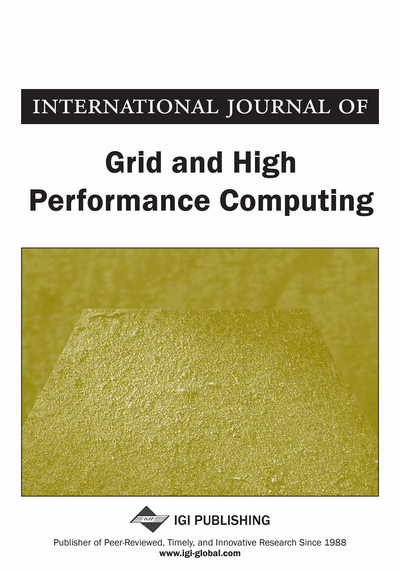# Reducing Inter-Process Communication Overhead in Parallel Sparse Matrix-Matrix Multiplication

Md Salman Ahmed (East Tennessee State University, Johnson City, TN, USA), Jennifer Houser (East Tennessee State University, Johnson City, TN, USA), Mohammad A. Hoque (East Tennessee State University, Johnson City, TN, USA), Rezaul Raju (University of Houston, Houston, TX, USA) and Phil Pfeiffer (East Tennessee State University, Johnson City, TN, USA)
DOI: 10.4018/IJGHPC.2017070104
Available
\$37.50
No Current Special Offers

## Abstract

Parallel sparse matrix-matrix multiplication algorithms (PSpGEMM) spend most of their running time on inter-process communication. In the case of distributed matrix-matrix multiplications, much of this time is spent on interchanging the partial results that are needed to calculate the final product matrix. This overhead can be reduced with a one-dimensional distributed algorithm for parallel sparse matrix-matrix multiplication that uses a novel accumulation pattern based on the logarithmic complexity of the number of processors (i.e., where is the number of processors). This algorithm's MPI communication overhead and execution time were evaluated on an HPC cluster, using randomly generated sparse matrices with dimensions up to one million by one million. The results showed a reduction of inter-process communication overhead for matrices with larger dimensions compared to another one dimensional parallel algorithm that takes run-time complexity for accumulating the results.
Article Preview
Top

## 1. Introduction

The widespread use and importance of matrix applications have created a compelling need for efficient algorithms for matrix-matrix multiplication. Matrix representations of real-world phenomena have numerous applications in science and technology, in fields that include electrical engineering, medical science, physics, quantum chemistry (VandeVondele et al., 2012), mathematics, and computer science. Matrix-matrix multiplication is indispensable for almost every research field that involves scientific computation and numerical methods like optimization, linear algebra, algebraic multigrid (Briggs et al., 2000), finite element analysis, and tensor contraction (Gilbert et al., 2008). In computer science, areas such as graphics, networking, wireless communication, video and audio analysis, image processing, graph theory (Dongen, 2008), big data analysis and language processing use matrix-matrix multiplication. Networks, for example, are commonly modeled with adjacency matrices: two-dimensional matrices whose elements represent connections and weights between a network’s nodes. Repetitive multiplication of adjacency matrices can determine multi-hop reachability, transitive closure and dynamic partitioning within a mobile ad hoc network.

Researchers have worked for several decades to devise matrix-matrix multiplication algorithms that outperform the traditional,algorithm. The need for such algorithms is driven by the processing of very large matrices, often with trillions of elements. Currently the fastest matrix-matrix multiplication algorithm, the Coppersmith-Winograd algorithm, has a run time complexity of(Williams, 2012). In computations involving matrices of larger dimensions, the main challenge for the matrix multiplication algorithm is a scarcity of computational resources. Increasingly, parallel processing is being used to address this challenge.

In one important special case, the nature of the data being processed creates particular opportunities for fast multiplication. Sparse matrices, or matrices whose elements consist largely of zeros, are commonly used to model real-world phenomena. Algorithms for sparse matrix-matrix multiplication improve on classic algorithms by focusing solely on products of nonzero elements. These algorithms’ performance depends on factors that include the number and distribution of nonzero elements in the matrices to multiply, the structures used to store the matrices, the number of processors allocated to a computation, and the efficiency of inter-processor coordination. In particular, the use of efficient communication models and data structures can greatly speed up parallel multiplication.

Over the past few decades, researchers have extensively studied the Parallel Sparse Generalized Matrix-Matrix multiplication problem, hereafter referred to as PSpGEMM (Buluc et al., 2008). Numerous algorithms have been designed that apply a variety of distribution models, storage mechanisms, and communication models to PSpGEMM. These approaches have been incorporated into standard libraries and tools such as BLAS. Despite all these efforts, however, the impact of inter-process communication cost on the overall speedup and scalability has received relatively little attention. The scalability of any PSpGEMM algorithm depends largely on its strategy for inter-process communication, due to the amount of communication needed to exchange partial results between processors during the compilation of the final product matrix.

## Complete Article List

Search this Journal:
Reset
Open Access Articles: Forthcoming
Volume 14: 6 Issues (2022): Forthcoming, Available for Pre-Order
Volume 13: 4 Issues (2021): 3 Released, 1 Forthcoming
Volume 12: 4 Issues (2020)
Volume 11: 4 Issues (2019)
Volume 10: 4 Issues (2018)
Volume 9: 4 Issues (2017)
Volume 8: 4 Issues (2016)
Volume 7: 4 Issues (2015)
Volume 6: 4 Issues (2014)
Volume 5: 4 Issues (2013)
Volume 4: 4 Issues (2012)
Volume 3: 4 Issues (2011)
Volume 2: 4 Issues (2010)
Volume 1: 4 Issues (2009)
View Complete Journal Contents Listing